## Visual Basic Program "VB MASS GAL.BAS": Mass of a Galaxy calculation based on rotation curve

### Introduction and Purpose

The reason of this program is the following question: How much dark matter ? The program is identical in performance as the QBASIC program:MASS_GAL.BAS

This program calculates:

• the mass of our Galaxy as a function of visible matter (Radius Visible Galaxy), the radius of the bulge (R bulge) and the speed of the Sun (SP velocity)
• the rotation speed of our Galaxy as a function of the distance from the center of our Galaxy. The maximum distance is 70000 lightyears.

Operation - part 1
When the program starts you get the following display: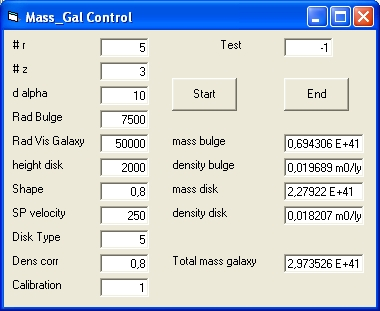The Mass Galaxy Control Form shows the following 11 parameters.
1. # r Defines the number of segments in the r direction. Standard value is 5.
2. # z Defines the number of segments in the z direction. Standard value is 3.
3. delta alpha Defines the delta angle alpha. Standard value is 10 degrees.
4. Radius BulgeDefines the radius of the bulge. Standard value = 7500 light years
5. Radius Visible galaxyDefines the radius of visible galaxy. Standard value = 60000 light years
6. Radius Total galaxyDefines the radius of total galaxy. Standard value = 70000 light years
7. height disk Defines the height of the disk at centre of Galaxy. Standard value is 2000 light years.
8. shape disk Defines the height of the disk at distance of Radius Total galaxy. Shape = 1 means that the distance is equal height disk. Standard value = 0.1
9. sp velocity Defines velocity setpoint for calibration at a distance of 25000 lightyears. Standard value is 250 km/sec.
10. disk type Defines the disk type.
There are 4 disk types:
1 = straight line. 2 = cosinus (0 - 90). 3 = cosinus (90 - 180). 4 = cosinus (0 - 180)
The standard value is 3.
11. disk Dens CorrectionDefines Disk Density Correction relative to bulge. Standard value is 0.6
12. calibration Defines calibration condition. 1 = yes. 0 = No. Standard value is 1.
The form has two commands START and END a special parameter called Test and there is a second Form called Display

Operation - part 2 START and END command
Just press START in order to start the simulation.

When the simulation is finished modify the parameters and press START again or press END to finish.

Operation - part 3 TEST parameter
There are 9 standard tests included which have the values from 1 to 9.

There is a special test with the value -1.

In order to select a test change the parameter Test to the requested value and select START
• When the value -1 is entered the 4 disk types are displayed: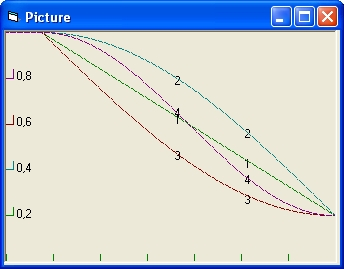• The following display shows the results for the test 1,2,3 and 4 in that order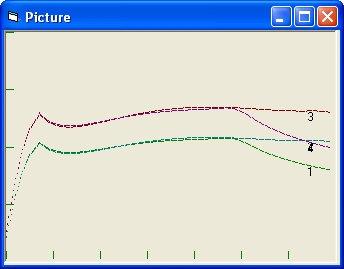Test Rad Vis SP vel height mass mass mass density density galaxy velocity disk bulge disk total bulge disk 1 50000 200 2000 0,423 1,795 2,218 0,0120 0,0107 2 70000 200 2000 0,420 2,648 3,069 0,0119 0,0111 3 70000 250 2000 0,652 4,105 4,757 0,0185 0,0173 4 50000 250 2000 0,655 2,278 3,436 0,0185 0,0165
• The following display shows the results for the test 2,5 and 6 in that order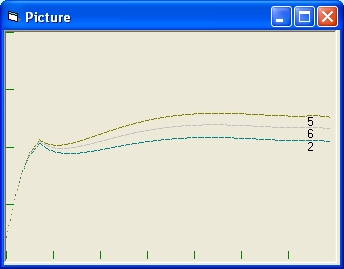Test SP vel height Density mass mass mass density density cali velocity disk correction bulge disk total bulge disk bration 2 200 2000 0,8 0,420 2,648 3,069 0,0119 0,0111 1 5 233,3 3000 0,8 0,420 3,973 4,393 0,0119 0,0111 0 6 218,4 2000 1,0 0,420 3,311 3,731 0,0119 0,0139 0
• The following display shows the results for the test 7,8 and 9 in that order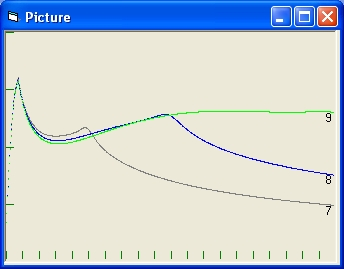Test SP vel radius Density mass mass mass density density cali velocity vis gal correction bulge disk total bulge disk bration 7 220 50000 0,18 1,143 1,561 2,705 0,0323 0,0059 1 8 214,7,3 100000 0,18 1,135 4,928 6,064 0,0321 0,0061 0 9 209,4 200000 0,18 1,118 12,068 13,187 0,0317 0,0066 0
Radius bulge = 7500, Radius Total Galaxy = 200000, Shape = 0,2
• The following display shows the results for the test 2,10 and 11 in that order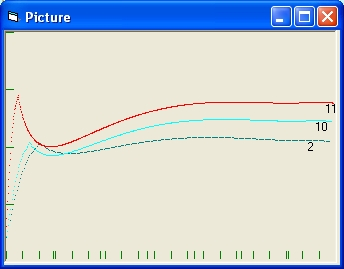Test Rad vis Rad tot SP height Density mass mass mass density density galaxy galaxy velocity disk correction bulge disk total bulge disk 2 70000 70000 200 2000 0,8 0,420 2,648 3,069 0,0119 0,0111 10 100000 100000 200 2000 0,8 0,432 5,3793 5,8118 0,0123 0,0114 11 200000 200000 200 1000 0,5 0,924 13,858 14,783 0,0262 0,0152
• The following display shows the results for the test 12 and 13 in that order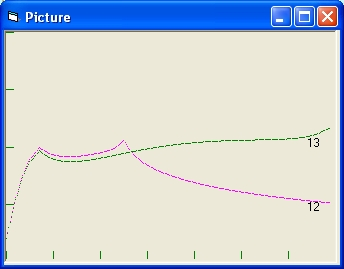Test Rad Vis SP vel height mass mass mass density density cali galaxy velocity disk bulge disk total bulge disk bration 12 26000 200 2000 0,381 0,627 1,0089 0,0108 0,0089 1 13 70000 187,1 2000 0,374 2,727 3,101 0,0106 0,0095 0
Radius bulge = 7500, Radius Total Galaxy = 70000, Shape = 0,3 Density Correction = 0.8

Test 12 and Test 13 are used to demonstrate the importance of the disk size i.e. the parameters Radius Visible galaxy and Radius Total galaxy. Specific the influence of mass outside the visible range.

• Test 12 is the target test. The parameter Radius Visible galaxy = 26000 and is much shorter as the parameter Radius Total galaxy. Calabration = 1
• Test 13 is the chalenge test. The parameter Radius Visible galaxy is much larger and equal to the parameter Radius Total galaxy. Calibration = 0
The mass within R = 26000 in both Test 12 and Test 13 is identical.
What the chalenge test shows that the mass outside R = 26000 influences the Galaxy Rotation Curve before R 26000.
• The following display shows the results for the test 14 and 15 in that order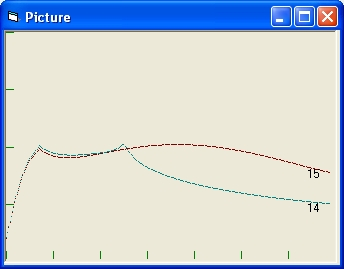Test Rad Vis SP vel height mass mass mass density density cali galaxy velocity disk bulge disk total bulge disk bration 14 26000 200 2000 0,388 0,5937 0,9824 0,0110 0,0092 1 15 70000 194,6 2000 0,381 1,644 2,026 0,0108 0,0115 0
Radius bulge = 7500, Radius Total Galaxy = 70000, Shape = 0 Density Correction = 0.8

Test 14 and Test 15 are used to demonstrate the importance of the disk size and shape i.e. the parameters Radius Visible galaxy , Radius Total galaxy and shape disk . Specific the influence of mass outside the visible range.

• Test 14 is the target test. The parameter Radius Visible galaxy = 26000 and is much shorter as the parameter Radius Total galaxy. Calabration = 1
• Test 15 is the chalenge test. The parameter Radius Visible galaxy is much larger and equal to the parameter Radius Total galaxy. Calibration = 0
The mass within R = 26000 in both Test 14 and Test 15 is identical.
What the chalenge test shows that the mass outside R = 26000 influences the Galaxy Rotation Curve before R 26000.

The difference between the Tests (12,13) and the Tests (14,15) is the parameter and shape disk.

• In the tests 12 and 13 the parameter shape disk is 0,3 . That means at the end of the disk the height of the disk is 0.3 * 2000. That means there is more mass outside this range.
• In the tests 12 and 13 the parameter shape disk is 0. That means at the end of the disk the height of the disk is 0.

## VB Program: VB MASS GAL.BAS source

In order to get the Visual Basic source and the executable program use the following zip file:VB MASS GAL.ZIP
This ZIP file contains the following files:
(1)VB Mass gal.vbw (2)VB Mass gal.vbp (3) VB Mass gal.exe (4) VB Mass gal.bas (5) VB Mass gal.frm and (6) VB Mass gal.pict.frm
The Visual Basic Executable program requires MSVDVM50.DLL: Msvdvm50.dll.zip

In order to retrieve the QBASIC source select:MASS_GAL.BAS
To see the QBASIC listing select:MASS_GAL.HTM
For the QBASIC Execution file select: MASS_GAL.EXE and: brun45.exe

For similar programs in Excel select circ11.xls to circ16.xls. The purpose of those programs is to calculate disk density profiles to support (flat) galaxy rotation curves.
For a description of those programs and of "Read me First" select circ11.xls.htm
For a program to calculate galaxy rotation curves use: Grotc.xls.
For a description of this programs and of "Read me First" select grotc.xls.htm

## Technical Data

For technical data see program2

## Reflection

The dark matter simulation gives 2 results:
1. When you compare the calculated rotation curve with a measured one (See the book: UNIVERSE ) page 492, you will see that the two are almost the same. This means that the amount of invisible matter or dark matter for our Galaxy is almost zero.
2. The real amount of visible mass of our Galaxy (taken the shape into account) is roughly four times as much as when you consider our Galaxy as a point.

Created: 9 September 1996
modified: 4 December 2001
Excel programs added: 1 Sept 2003
Visual Basic Program added: 1 May 2011